# Math in Focus Grade 6 Chapter 4 Lesson 4.1 Answer Key Comparing Two Quantities

Go through the Math in Focus Grade 6 Workbook Answer Key Chapter 4 Lesson 4.1 Comparing Two Quantities to finish your assignments.

## Math in Focus Grade 6 Course 1 A Chapter 4 Lesson 4.1 Answer Key Comparing Two Quantities

### Math in Focus Grade 6 Chapter 4 Lesson 4.1 Guided Practice Answer Key

Complete.

Question 1.
The ratio of the number of CDs to the number of CD sleeves is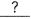:.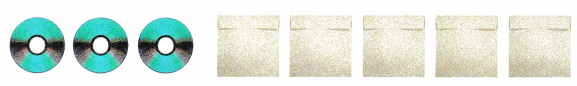3 : 5
Explanation:
The number of CDs = 3
The number of sleeves = 5
the ratio of CDs = 3:5

Question 2.
Mrs. Carter buys 4 bags of apples and 7 bags of oranges. Each bag has an equal number of fruits.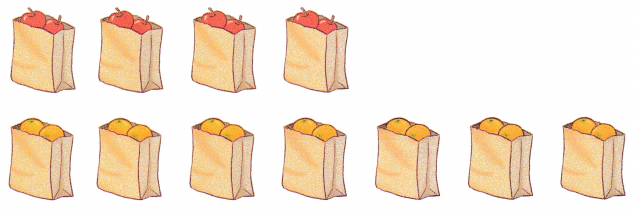The ratio of the number of apples to the number of oranges is:.
4 : 7
Explanation:
The number of apples = 4
The number of oranges = 7
The ratio of the number of apples to the number of oranges is 4:7

Question 3.
Desiree has a cat that weighs 12 pounds and a dog that weighs 13 pounds.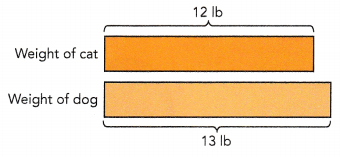a) The ratio of the weight of the cat to the weight of the dog is:.
12 : 13
Explanation:
The weight of cat = 12lb
The weight of dog = 13lb
The ratio of the weight of the cat to the weight of the dog is =12:13

b) The ratio of the weight of the dog to the weight of the cat is:.
13 : 12
Explanation:
The weight of cat = 12lb
The weight of dog = 13lb
The ratio of the weight of the dog to the weight of the cat is = 13:12

State whether each of the following can be expressed as a ratio.

Question 4.
6 kg and 84 kg
yes, expressed as 1 : 14
Explanation:
The ratio of 6 kg and 84kg is expressed as
= 6 : 84
=1 : 14

Question 5.
72 ft and 2 yd
yes, expressed as 1 : 12
Explanation:
The ratio of  72ft and 2yds
To express in ration we should convert the both the numbers in one unit( Either in feet of yards) as shown below
(2yd = 6ft)
= 6 : 72
= 1 : 12

Question 6.
3 oz and 30 in.
yes, expressed as 1 : 6
Explanation:
The ratio of 3 oz and 30 in.
3 oz = 5in
3 oz = 5.2 in
= 5 : 30
= 1:6

Complete.

Question 7.
13 cm : 2 m = 13 cm :cm Think: 1 m = 100 cm, so 2 m =cm.
=:.
13 : 200
Explanation:
13 cm : 2 m = 13 cm : 200 cm
Think: 1 m = 100 cm,
so 2 m = 200 cm.
= 13 : 200.

Question 8.
5 kg : 13 g =g :g Think: 1 kg = 1,000 g, so 5 kg =g.
=:.
5000 : 13
Explanation:
5 kg : 13 g = 5000 g : 13 g
Think: 1 kg = 1,000 g,
so 5 kg = 5000 g.
5000 : 13

Question 9.
9 mL : 7 L =mL :mL Think: 1 L = 1,000 mL, so 7 L =mL.
=:.
9 : 7000
Explanation:
9 mL : 7 L = 9 mL : 7000 mL
Think: 1 L = 1,000 mL,
so 7 L = 7000 mL.
= 9 : 7000

Question 10.
John keeps 17 angelfish and 24 guppies.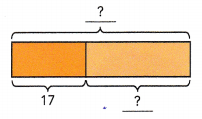a) Find the ratio of the number of angelfish to the number of guppies.
The ratio of the number of angelfish to the number of guppies is:.
17 : 24
Explanation:
John keeps 17 angelfish and 24 guppies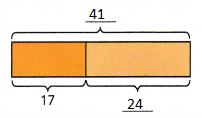The ratio of the number of angelfish to the number of guppies is 17 : 24

b) Find the ratio of the number of guppies to the total number of fish.
Total number offish =+=The ratio of the number of guppies to the total number of fish is 24 : 41.
24 : 58
Explanation:
John keeps 17 angelfish and 24 guppies
Total number offish = 17 + 41 = 58
The ratio of the number of guppies to the total number of fish is 24 : 58.

Question 11.
Alex has a string 72 centimeters long. He cuts it into two pieces. The length of the shorter piece is 31 centimeters. What is the ratio of the length of the longer piece to the total length of the string?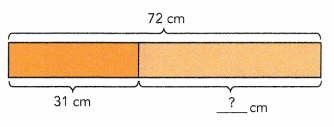72 –=The length of the longer piece iscentimeters,cm :cm =:The ratio of the length of the longer piece to the total length of the string is:.
41 : 72
Explanation: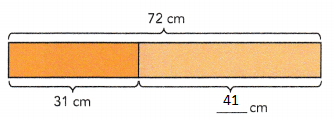72 – 31 = 41
The length of the longer piece is 41 centimeters,
31 cm : 41 cm = 31 : 41
The ratio of the length of the longer piece to the total length of the string is 41 : 72.

Question 12.
The total amount of money Pamela and Rachel saved in a week was $45. Pamela saved$13.
a) Find the amount of money Rachel saved.
$32 Explanation: The total amount of money Pamela and Rachel saved in a week was$45
Pamela saved $13.$45 – $13 =$32
the amount of money Rachel saved = $32 b) Find the ratio of the total amount of money Pamela and Rachel saved to the amount of money Rachel saved. Answer: 4 : 9 Explanation: The total amount of money Pamela and Rachel saved in a week was$45
The amount of money Rachel saved = $32 The ration of the total amount of money Pamela and Rachel saved to the amount of money Rachel saved is 32 : 72 4 : 9 (8 x 4 = 32 and 8 x 9 = 72) Complete. You may draw a model to help you. Question 13. The ratio of the number of white parrots to the number of green parrots is 7 : 8. Write a ratio to describe the number of green parrots to the total number of parrots. Total number of parrots =+=units The ratio of the number of green parrots to the total number of parrots is:or $$\frac{?}{?}$$. Answer: 8 : 15 Explanation: The ratio of the number of white parrots to the number of green parrots is 7 : 8. Total number of parrots = 7 + 8 = 15 units The ratio of the number of green parrots to the total number of parrots is 8 : 15 or $$\frac{8}{15}$$. Solve. You may draw a model to help you. Question 14. The ratio of the number of male teachers to the number of female teachers in a school is 5 : 9. a) What fraction of the teachers are females? Answer: $$\frac{9}{14}$$. b) What fraction of the teachers are males? Answer: $$\frac{5}{14}$$. Explanation: The ratio of the number of male teachers to the number of female teachers in a school is 5 : 9. Total teachers = 5 + 9 = 14 fraction of male teachers = $$\frac{9}{14}$$. fraction of female teachers = $$\frac{5}{14}$$ Complete. Question 15. The height of a coconut tree is 48 feet and the height of a papaya tree is 12 feet. a) How many times the height of the papaya tree is the height of the coconut tree?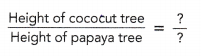The height of the coconut tree istimes the height of the papaya tree. Answer: 4ft Explanation: The height of a coconut tree is 48 feet and the height of a papaya tree is 12 feet. The height of the coconut tree is 4 times the height of the papaya tree.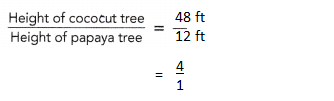b) How many times the height of the coconut tree is the height of the papaya tree?The height of the papaya tree istimes the height of the coconut tree. Answer: $$\frac{1}{4}$$. Explanation: The height of a coconut tree is 48 feet and the height of a papaya tree is 12 feet. The height of the papaya tree is 1/4 times the height of the coconut tree. ### Math in Focus Course 1A Practice 4.1 Answer Key Write two ratios to compare the quantities. Question 1.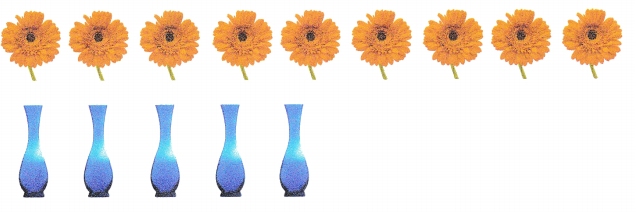Answer: 9 : 5 Explanation: there are 9 sun flowers there are 5 flower vases the ratio of flowers and vases are 9:5 Question 2. Catherine has 23 video game disks and Dylan has 37 video game disks.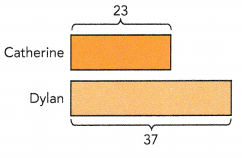Answer: 23 : 37 Explanation: Catherine has 23 video game disks and Dylan has 37 video game disks. Ratio of the both video games = 23 : 37 Question 3. In a school, there are 8 classes in the sixth grade and 7 classes in the seventh grade. Each class has an equal number of students.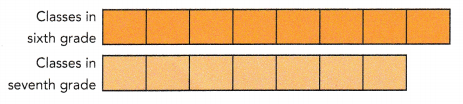Answer: 8 : 7 Explanation: In a school, there are 8 classes in the sixth grade and 7 classes in the seventh grade. Each class has an equal number of students Ratio of the students = 8 : 7 State whether each of the following can be expressed as a ratio. Question 4. 6 cm and 60 g Answer: No, it can’t be expressed in ratio. Explanation: Both the quantities must be of same units, here both are different in units, which can not be compared. Question 5. 54 kg and 54 m Answer: No, it can’t be expressed in ratio. Explanation: Both the quantities must be of same units, here both are different in units, which can not be compared. Question 6. 12 g and 45 kg Answer: Yes, it can be expressed in ratio. Explanation: Both the quantities must be of same units. 45Kg = 45 x 1000 = 45000g 1kg = 1000g 12 : 45000 Question 7. 87 ft and 93 yd Answer: Yes, it can be expressed in ratio. Explanation: Both the quantities must be of same units. 1yd = 3ft 93 x 3 = 279 ft 87 ft : 279 ft 29 : 93 Solve. Show your work. Question 8. There are 123 students in a drama club. 65 of them are girls. a) What is the ratio of the number of boys to the number of girls? Answer: 58 : 65 Explanation: There are 123 students in a drama club. 65 of them are girls. number of boys 123 – 65 =58 the ratio of the number of boys to the number of girls = 58 : 65 b) What is the ratio of the number of boys to the total number of students? Answer: 58 : 123 Explanation: There are 123 students in a drama club. 65 of them are girls. the number of boys 123 – 65 =58 the ratio of the number of boys to the total number of students = 58 : 123 Question 9. There were 37 clarinet players and 150 trumpet players who tried out for a music competition.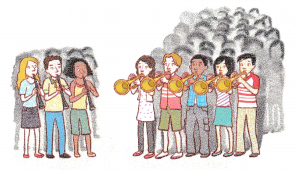a) Find the ratio of the number of clarinet players to the number of trumpet players. Answer: 37 : 150 Explanation: There were 37 clarinet players and 150 trumpet players who tried out for a music competition. The ratio of the number of clarinet players to the number of trumpet players = 37 : 150 b) Find the ratio of the number of trumpet players to the total number of players. Answer: 150 : 187 Explanation: There were 37 clarinet players and 150 trumpet players who tried out for a music competition. the total number of players = 37 + 150 = 187 the ratio of the number of trumpet players to the total number of players = 150 : 187 Question 10. CD Carl had$43 when he entered the museum gift shop. After spending some money, he had $18 left. a) Find the amount of money Carl spent. Answer:$25
Explanation:
CD Carl had $43 he had$18 left.
He spend money 43 – 18 = 25

b) Find the ratio of the amount of money Carl spent to the amount of money he had when he entered the gift shop.
25 : 43
Explanation:
CD Carl had $43 he spend$25.
the ratio of the amount of money Carl spent to the amount of money he had 25 : 43

Question 11.
Kelvin’s monthly allowance is $42 and Mike’s monthly allowance is$63. How many times Mike’s monthly allowance is Kelvin’s monthly allowance?
$$\frac{3}{2}$$.
Explanation:
Kelvin’s monthly allowance is $42 Mike’s monthly allowance is$63
$$\frac{63}{42}$$ i.e., { 21 x 2 = 42 & 21 x 3 = 63}

$$\frac{3}{2}$$

Question 12.
The ratio of the weight of vegetables sold to the weight of fruits sold is 45 : 144.
a) How many times the weight of vegetables sold is the weight of fruits sold?
$$\frac{45}{144}$$

b) What fraction of the total weight of vegetables and fruits sold is the weight of vegetables sold?
$$\frac{144}{189}$$
Explanation:
The ratio of the weight of vegetables sold to the weight of fruits sold is 45 : 144.
Total vegetables and fruits = 144 + 45 = 189
number of times the weight of vegetables sold is the weight of fruits sold
= $$\frac{45}{144}$$
fraction of the total weight of vegetables and fruits sold is the weight of vegetables sold
= $$\frac{144}{189}$$

Question 13.
The ratio of the length to the width of a rectangle is 5 : 2.
a) Express the difference between the length and the width of the rectangle as a fraction of the length of the rectangle.
The difference is 3
Explanation:
The ratio of the length to the width of a rectangle is 5 : 2.
difference between width and length
= 5 – 2 = 3
b) Express the width of the rectangle as a fraction of the perimeter of the rectangle.
$$\frac{1}{7}$$
Explanation:
The ratio of the length to the width of a rectangle is 5 : 2.
difference between width and length
= 5 – 2 = 3
perimeter = $$\frac{2}{14}$$
$$\frac{1}{7}$$

Math Journal Describe a situation that each ratio could represent.

Question 14.
5 : 16
5 : 16
Number of base ball players : Number of foot ball players
Explanation:
The ratio of Baseball players and Football players are 5 : 16

Question 15.
98 : 3